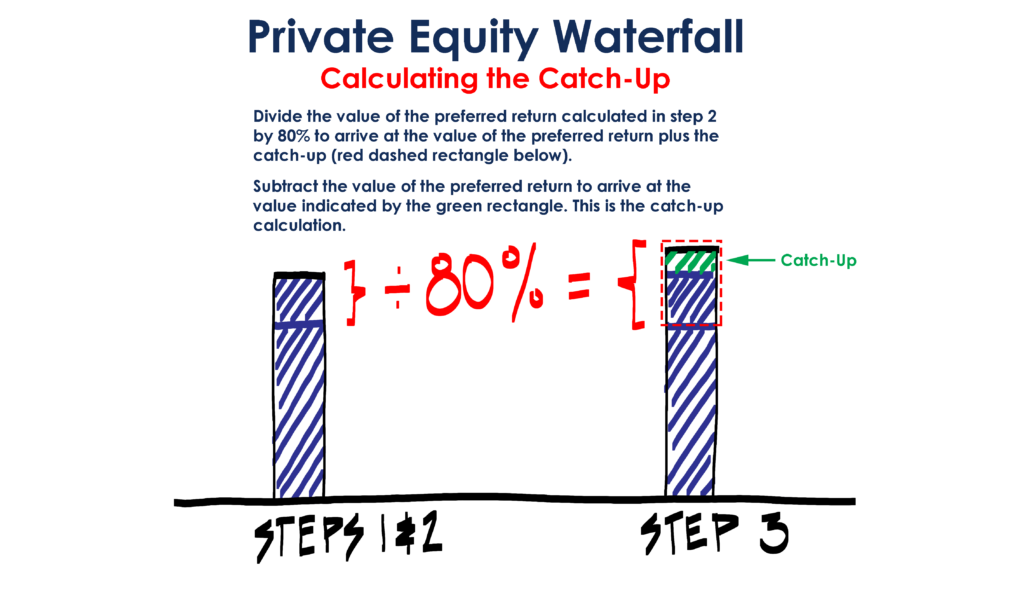Select Page

# Private Equity Catch Up Calculation

• Peter Lynch

The calculation behind the catch-up provision that determines the general partner’s (GP) carried interest at a private equity fund can cause some confusion. In this post we will explain the math in the Excel template available on ASM.

For reference, the calculation refers to the second example cited in this Excel template: Distribution Waterfall with 5 Examples (related post). The language for the second example cited in the Excel file is as follows:

First, 100% of all cash inflows to the LP until the cumulative distributions equal the original capital invested plus some preferred return.

Second, a “20% catch up” to the GP equivalent to 20% of the of the distributions realized in step 1 plus the distributions realized in this step.

Third, thereafter, cash flows in excess of distributions made in step 1 and step 2 (if any) are distributed 80% to the LP and 20% to the GP.Based on the emails I receive, most of the confusion comes from the calculation used in the second step. So let’s reframe the objective: In step 2 you want the GP to receive 20% of all distributions up to and including step 2. For this exercise, think about (All Distributions up to and including Step Two) as all cash flows received by both the GP and LP up to and including Step Two.

If the GP is supposed to get 20% of (All Distributions up to and including Step Two), it follows that the LP has received 80% of (All Distributions up to Step Two):

(All Distributions up to and including Step Two) * 0.8 = (LP First Distribution)

(All Distributions up to and including Step Two) = (LP First Distribution) /0.8

And since the GP only receives what is not allocated to the LP (what the GP receives = the catch up):

(Catch Up) = (LP First Distribution) /0.8 – (LP First Distribution)

If subtracting the “(LP First Distribution)” is confusing, think about it this way (different formula, same result):

(Catch Up) = ((LP First Distribution) /0.8)*0.2

To help this sink in I thought I would provide an additional way to think through this exercise: The Catch Up is equal to 20% of all cash flows received in both steps 1 and 2. It follows that:

C = Catch Up

P = LP return in First Distribution

C = 0.2*P + 0.2*C

0.8*C = 0.2*P

C = P*0.2/0.8

C = P * 0.25

For the exercise I thought the first approach would make it easier to follow the formulas (I find the 0.25 in the second formula has the potential to be confusing), but generally multiple examples help.

Learn more about private equity transactions with ASM’s Private Equity Training course. The Private Equity Training course was developed by industry professionals. The content goes beyond the LBO model to explain how private equity professionals source, structure and close transactions.